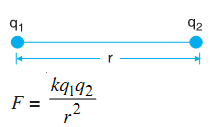# Electrostatic Force

The Coulomb’s Law governs the force between the charged particles. It was first studied by a French physicist Charles Augustine de Coulomb. Coulomb presented the inference of his experiments in the form of a law which is called Coulomb's law.

According to Coulomb's law, the magnitude of the force of attraction (or repulsion) between two point charges is directly proportional to the product of the quantity of two charges and inversely proportional to the square of the distance between them.

If a charge, q1 is placed at a distance, r from a similar charge q2 the two charges will continue to repel each other with a force:where k is a constant of proportionality depending upon the nature of the medium in which the charges are placed.

k = 9 × 109 N m2 C-2 for vacuum (or air).

Charge is a scalar quantity. Coulomb is a SI unit of charge represented by C.

If q1 = q2 = 1 C, r = 1 m,

F = 9 x 10-9 N

Thus, 1 C is the charge when placed at a distance of 1 m from an equal like charge in vacuum, experiences a repulsive force of 1 N.

Force is directed along the line joining the centres of the two charges. For like charges, force is repulsive (positive in sign), while for unlike charges it is attractive (with negative sign).